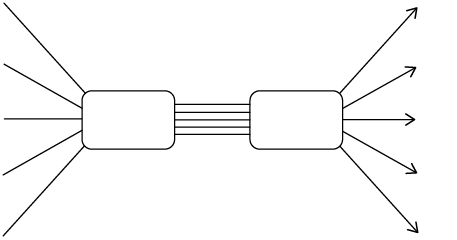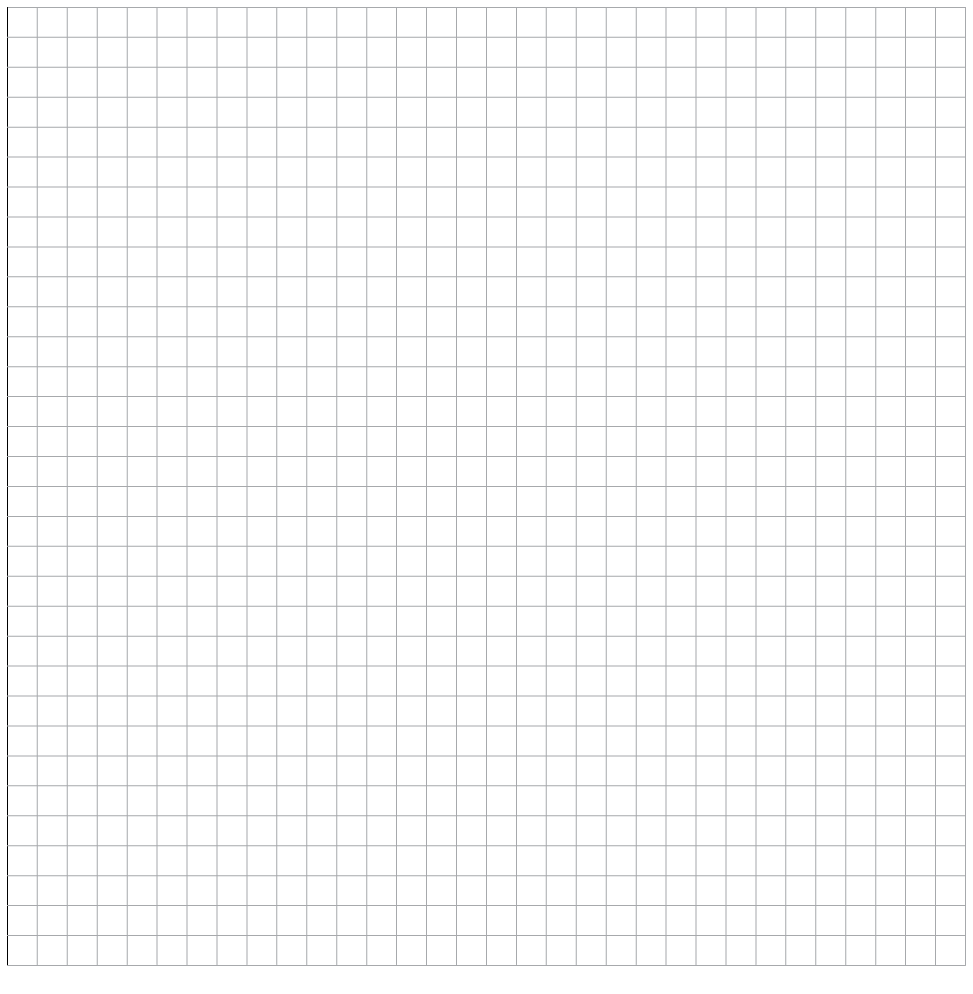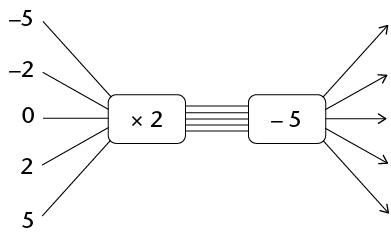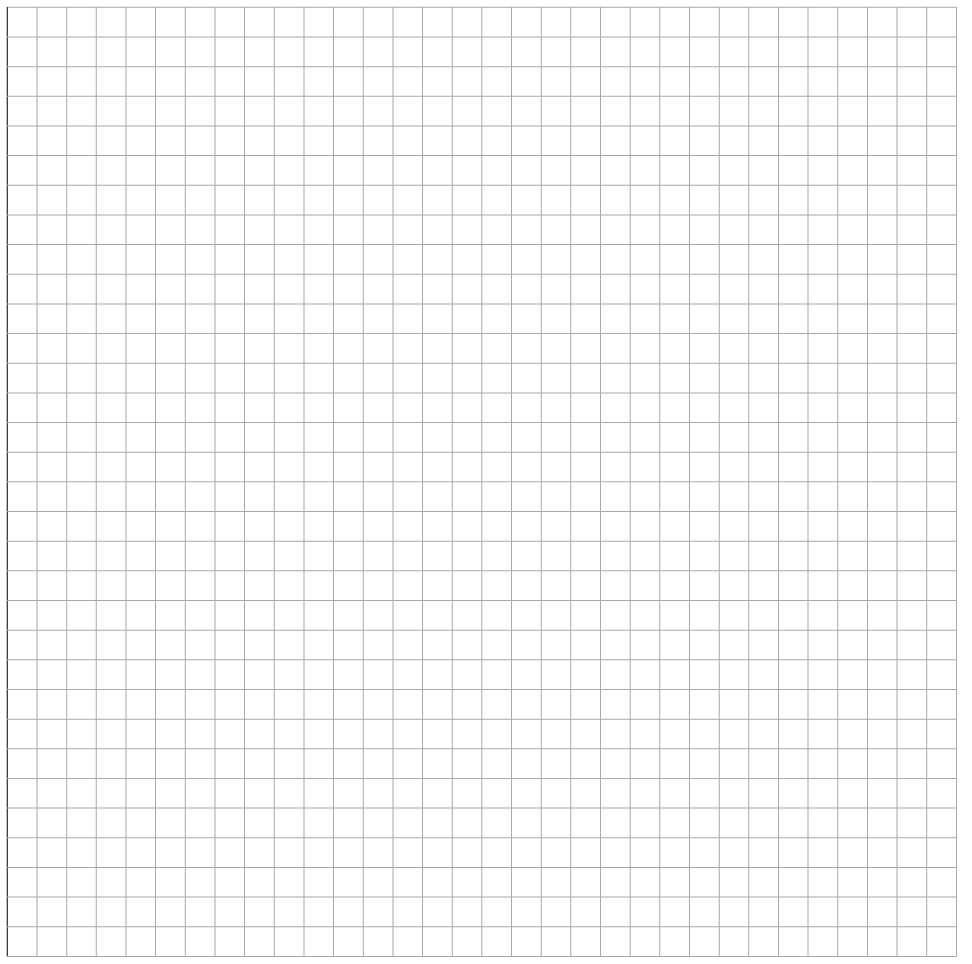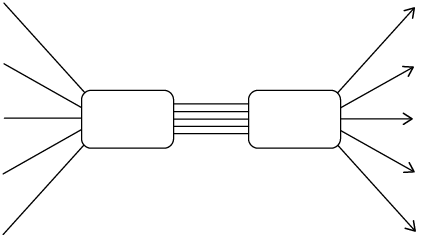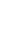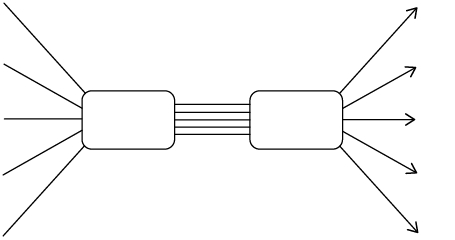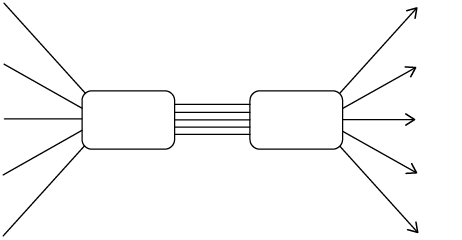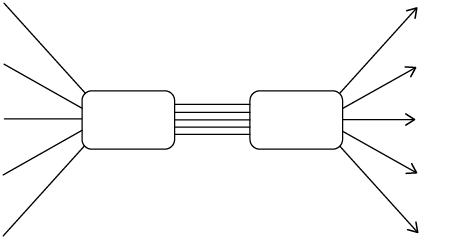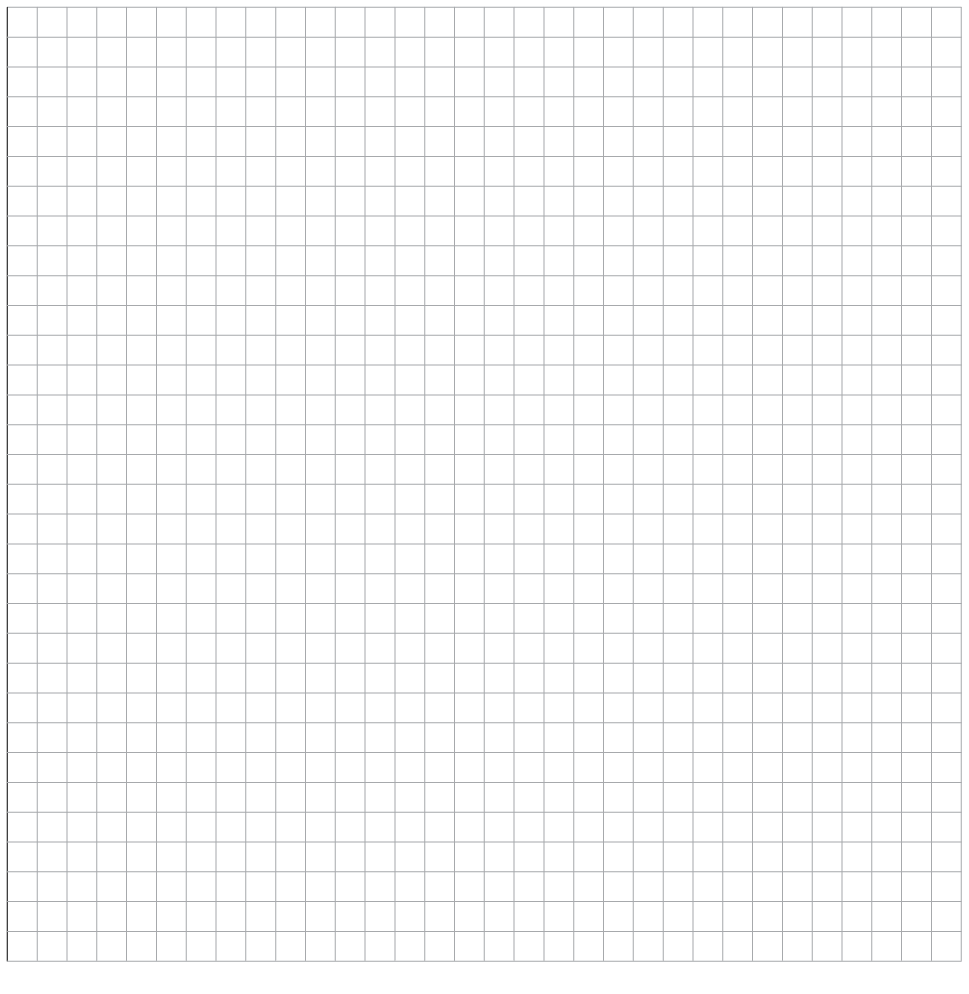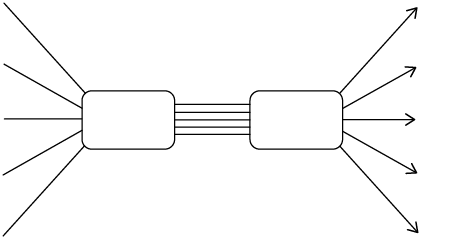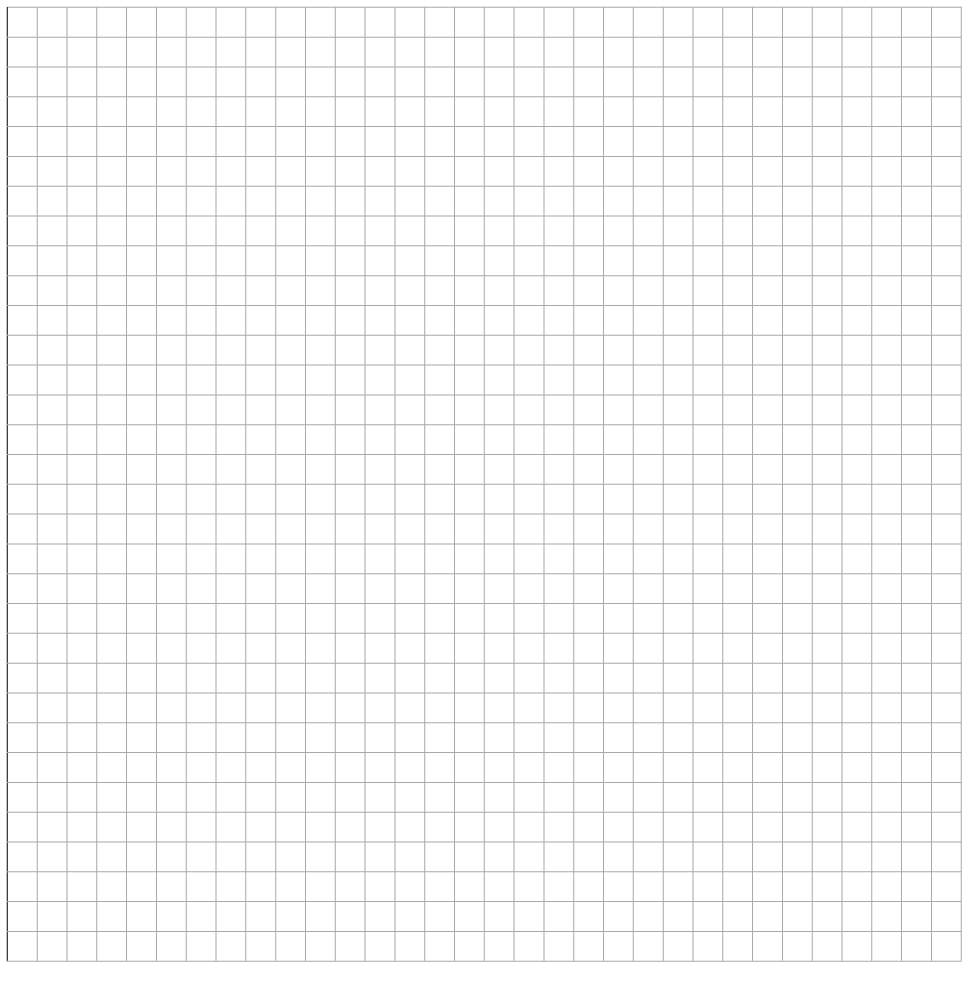• You are going to choose a number, multiply it by 5, and subtract the answer from 50.

1. Choose any number from set A and do the above calculations.

2. Choose any number from set B and do the above calculations.

3. If you choose any other number from set B, do you think the answer will also be a negative number?

1. Write down all the different output numbers that will be obtained when the calculations $50 - 5x$ are performed on the different numbers in set A.

Output numbers are numbers that you obtain when you apply the rule to the input numbers.

2. Write down the output numbers that will be obtained when the formula $50 - 5x$ is applied to set B.

1. Complete the following table for set A:

 Input numbers 1 2 3 4 5 6 7 8 9 Values of $50 - 5x$
2. Complete the following table for set B:

 Input numbers 20 30 40 50 60 70 80 90 Values of $50 - 5x$
• In this question your set of input numbers will be the even numbers 2; 4; 6; 8; 10; ...

1. What will all the output numbers be if the rule $2n + 1$ is applied to the set of even numbers? Write a list.

2. What will the output numbers be if the rule $2n- 1$is applied?

3. What will the output numbers be if the rule $2n + 5$ is applied?

4. What will the output numbers be if the rule $3n + 1$ is applied?

1. What kind of output numbers will be obtained by applying the rule $x - 1 000$ to natural numbers smaller than 1 000?

2. What kind of output numbers will be obtained by applying the rule $\frac{x}{10} + 10$ to natural numbers smaller than 10?

3. If you use the rule $30x + 2$, and use input numbers that are positive fractions with denominators 2, 3 and 5, what kind of output numbers will you obtain?

• A quantity that changes is called a variable quantity or just a variable.

If one variable quantity is influenced by another, we say there is a relationship between the two variables. You can sometimes work out which number is linked to a specific value of the other variable.

The output number can also be called the output value, or the value of the expression, which is $10x + 5$ in this case.

Arelationship between two variables in which there is only one output number for each input number, is called a function.

• With a table that shows some values of the two variables. A table shows clearly which value of the output variable corresponds to each particular value of the input variable.
• A flow diagram, which shows what calculations are to be done to calculate the output number that corresponds to a given input variable.
• A formula, which also describes what calculations are to be done to calculate the output number that corresponds to a given input variable.
• A graph.
• Complete the flow diagram: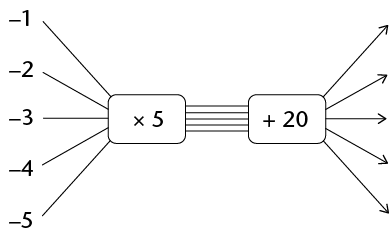A completed flow diagram shows two kinds of information:

• It shows what calculations are done to produce the output numbers.
• It shows which output number is connected to which input number.
• Each input number is multiplied by 5, then 20 is added, to produce the output numbers.
• Which output numbers correspond to which input numbers.
• The output numbers of a function are also called function values. Hence the formula can also be written as

function value = $5x + 20$

• Complete this table for the function described by $5x + 20$:

 Input numbers -1 -2 -3 -4 -5 Function values
• Draw a graph of this function below.• A graph of a certain function is given below. Complete the table for this function.

 Input numbers Function values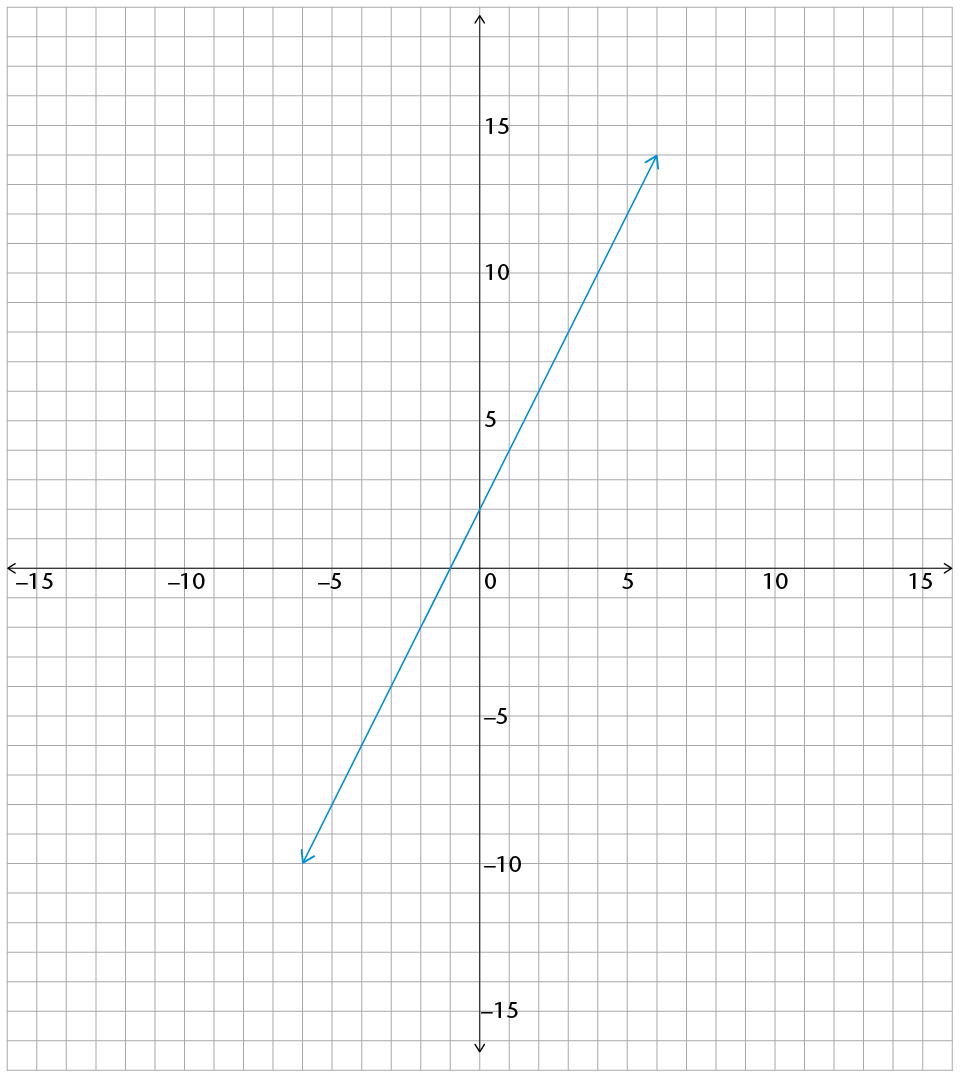• a flow diagram

• a table of values for the set of integers from -5 to 5

• a graph

• The relationship described by the expression $3x + 4$

• The relationship described by the expression $2x - 5$

• The relationship described by the expression $\frac{1}{2}x +2$

• The relationship described by the expression $-3x + 4$

• The relationship described by the expression $2,5x + 1,5$

• The relationship described by the expression $0,2x + 1,4$

• The relationship described by the expression $-2x-4$

•